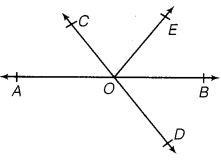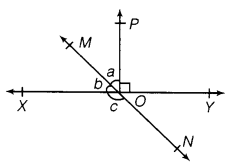NCERT Solutions for Class 9 Maths Chapter 6 Lines and Angles Ex 6.1

# NCERT Solutions for Class 9 Maths Chapter 6 Lines and Angles Ex 6.1

## NCERT Solutions for Class 9 Maths Chapter 6 Lines and Angles Ex 6.1

NCERT Solutions for Class 9 Maths Chapter 6 Lines and Angles Ex 6.1 are the part of NCERT Solutions for Class 9 Maths. Here you can find the NCERT Solutions for Chapter 6 Lines and Angles Ex 6.1.

Ex 6.1 Class 9 Maths Question 1.
In the figure, lines AB and CD intersect at O. If AOC + BOE = 70° and BOD = 40°, find BOE and reflex COE.Solution:
Here,

AOC and BOD are vertically opposite angles.
AOC = BOD
AOC =  40°                   [BOD  = 40°    (Given)]      …(i)
We have,
AOC + BOE = 70°       (Given)
40° +
BOE = 70°               [from eq.(i)]
BOE = 30°
Also,
AOC + COE + BOE = 180°        (Linear pair of angles)
40° + COE + 30° = 180°

⇒ ∠COE = 110°
Now,
COE + reflex COE = 360°     (Angles at a point)
110° + reflex
COE = 360°
Reflex COE = 250°

Ex 6.1 Class 9 Maths Question 2.
In figure, lines XY and MN intersect at O. If
POY = 90° and a : b = 2: 3, find c.Solution:
We have,

POY = 90°
POY + POX = 180°          (Linear pair of angles)
POX = 90°
a + b = 90°      ……….(i)
Also, a : b = 2 : 3        (Given)
Let a = 2k and b = 3k
Now, from Eq. (i), we get
2k + 3k = 90°

5k = 90°

k = 18°
a = 2 × 18° = 36° and b = 3 × 18° = 54°
Now,
MOX + XON = 180°           (Linear pair of angles)

b + c = 180°
54° + c = 180°
c = 126°

Ex 6.1 Class 9 Maths Question 3.
In the given figure,
PQR = PRQ, then prove that PQS = PRT.

Solution:
PQS + PQR = 180°      [Linear pair of angles] …………(i)
PRT + PRQ = 180°      [Linear pair of angles] …………(ii)
From equations. (i) and (ii), we have
PQS + PQR = PRT + PRQ
PQS = PRT      [ PQR = PRQ    (given)]

Ex 6.1 Class 9 Maths Question 4.
In the given figure, if x + y = w + z, then prove that AOB is a line.

Solution:
We know that the sum of all the angles at a point = 360°

x + y + z + w = 360°

or, (x + y) + (z + w) = 360°
But (x + y) = (
z + w)             [Given]
(x + y) + (x + y) = 360°
2(x + y) = 360°
or, (x + y) = 360°/2 = 180°
AOB is a straight line.

Ex 6.1 Class 9 Maths Question 5.
In figure, POQ is a line. Ray OR is perpendicular to line PQ. OS is another ray lying between rays OP and OR. Prove that ROS = ½ (QOS – POS)

Solution:
We have, POQ is a straight line.     [Given]
POS + ROS + ROQ = 180°
But OR
PQ,
ROQ = 90°
POS + ROS + 90° = 180°
POS + ROS = 90°
ROS = 90° – POS      … (1)
Now, we have
ROS + ROQ = QOS
ROS + 90° = QOS
ROS = QOS – 90°      …(2)

Adding (1) and (2), we get
2
ROS = (QOS – POS)
ROS = ½ (QOS POS)

Ex 6.1 Class 9 Maths Question 6.
It is given that XYZ = 64° and XY is produced to point P. Draw a figure from the given information. If ray YQ bisects ZYP, find XYQ and reflex QYP.

Solution:
We have, XYP is a straight line.

XYZ + ZYQ + QYP = 180°
64° + ZYQ + QYP = 180°         [ XYZ = 64°  (given)]
64° + 2QYP = 180°                     [YQ bisects ZYP so, QYP = ZYQ]

2QYP = 180° – 64° = 116°
QYP = 116°/2 = 58°
Reflex QYP = 360° – 58° = 302°
Since
XYQ = XYZ + ZYQ
XYQ = 64° + QYP                     [∵ ∠XYZ = 64° (Given) and ZYQ = QYP]
XYQ = 64° + 58° = 122°             [QYP = 58°]
Thus,
XYQ = 122° and reflex QYP = 302°.

NCERT Solutions for Maths Class 10

NCERT Solutions for Maths Class 12